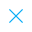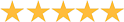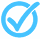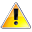+91-85588-96644

Keep me logged in
You can't leave Captcha Code empty
By submitting this form, you agree to the Terms & Privacy Policy.
ORTests given# Coordinate Geometry - 1 (Class IX) By TCY All tests

5.0Questions

Time

Highest
score

Level

## ENGLISH

Language

Topics Covered:Graphs of Linear EquationsPoints in a PlaneLinear Equation Definition and GraphsLinear Equations with One and Two VariablesLocation of PointsPair of Linear Equationsin 2 VariablesCo-ordinate GeometryGraph of a Linear Equation in 2 VariablesEquation of Lines Parallel to Axes